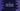# fabs function in C with example# fabs function in C :

The fabs function is defined in math.h header file. It is used to find out the absolute value of a number. fabs takes one double number as argument and returns the absolute value of that number. In this tutorial , we will show you how to use fabs function in C with examples.

## Syntax of fabs :

The syntax of fabs() is as below :

``double fabs(double n)``

As you can see that it takes one double parameter n and returns its absolute value as double.

Always remember to pass one double number to this function. If you want to find the absolute value for an integer or floating point number, first convert it to a double.

## Example of using fabs :

Now let’s try to find the absolute values for a set of numbers using fabs :

``````#include <stdio.h>
#include <math.h>

int main()
{
double n;

n = 12;
printf("The absolute value of %.2f is %.2f\n",n,fabs(n));

n = -12;
printf("The absolute value of %.2f is %.2f\n",n,fabs(n));

n = 11.563;
printf("The absolute value of %.3f is %.3f\n",n,fabs(n));

n = -1.012;
printf("The absolute value of %.3f is %.3f\n",n,fabs(n));

n = -0.44;
printf("The absolute value of %.2f is %.2f\n",n,fabs(n));

return 0;
}``````

The output of the above program is :

``````The absolute value of 12.00 is 12.00
The absolute value of -12.00 is 12.00
The absolute value of 11.563 is 11.563
The absolute value of -1.012 is 1.012
The absolute value of -0.44 is 0.44``````

You might also like :

### Where is the color and why codevscolor ?

Long story short, I love paintings and I paint on weekends. We(me and my wife) have one Youtube channel. Don't forget to subscribe ❤️❤️🤩